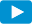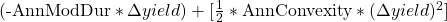Notes for Level I CFA® Program

R54 Understanding Fixed-Income Risk and Return

4.1. Yield VolatilityWatch VideoIf there is a change in a bond’s YTM, there will be a corresponding change in the price of a bond. The change in the price can be explained as the product of two factors:

1. Price value of a basis point (PVBP): Impact on bond price of a one basis point change in YTM. This factor is based on the duration and convexity of the bond.
2. Number of basis points: This is the change in yield measured in basis points.

The percentage change in the price of a bond for a given change in yield can also be determined using this equation:

%ΔPVFULL =Example 17: Ranking bonds in terms of interest rate risk

A fixed-income analyst is asked to rank three bonds in terms of interest rate risk. The increases in the yields-to-maturity represent the “worst case” for the scenario being considered.

 Bond Modified Duration Convexity ∆Yield A 3.65 14.8 50 bps B 5.75 38.7 25 bps C 12.28 146 15 bps

The modified duration and convexity statistics are annualized. ∆Yield is the increase in the annual yield-to-maturity. Rank the bonds in terms of interest rate risk.

Solution:

Calculate the estimated price change for each bond:

Bond A:

The duration effect is -3.65 × 0.005 = -1.825%.

The convexity effect is 0.5 × 14.8 × 0.005^2= 0.0185%.

The expected change in bond price is -1.825% + 0.0185% = -1.8065%.

Bond B:

The duration effect is -5.75 × 0.0025 = -1.4375%.

The convexity effect is 0.5 × 38.7 × 0.0025^2= 0.0121%.

The expected change in bond price is -1.4375% + 0.0121% = -1.4254%.

Bond C:

The duration effect is -12.28 × 0.0015 = -1.842%.

The convexity effect is 0.5 × 146 × 0.0015^2= 0.0164%.

The expected change in bond price is -1.842% + 0.0164% = -1.8256%.

Bond C has the highest degree of interest rate risk (a potential loss of 1.8256%), followed by Bond A (a potential loss of 1.8065%) and Bond B (a potential loss of 1.4254%).